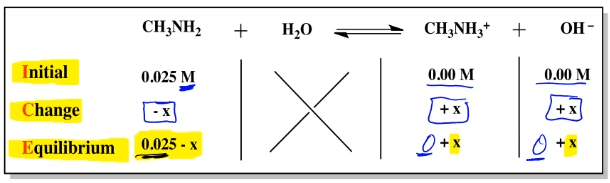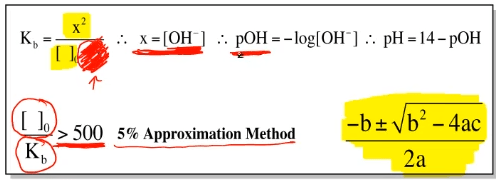Clutch Prep is now a part of Pearson
Ch.15 - Acid and Base EquilibriumWorksheetSee all chapters

# Weak Bases

See all sections
Sections
Identifying Acids and Bases
Arrhenius Acid and Base
Bronsted Lowry Acid and Base
Amphoteric
Lewis Acid and Base
pH and pOH
Ka and Kb
Ionic Salts
Diprotic Acid
Polyprotic AcidJules Bruno

# Weak bases

In this guide, we’ll talk about how to calculate the pH of a weak base solution. First of all, a weak base, all weak bases in fact, have a base association constant or Kb less than 1.

## Calculating pH of a weak base solution

In order to find the pH of a weak base solution, you’re going to have to set up an ICE chart. Remember, ICE stands for Initial Change Equilibrium. The units inside the ICE chart for the weak base will be in molarity. Remember, that’s just moles over liters. The initial amounts of your products will be zero. Then remember the changes we lose reactants in order to make products. Here we’re going to have -x from our reactant and our products are being formed so that’s +x and +x. We’re going to say here that equilibrium is just initial minus the change here so that’s 0.025 - x. Here this is 0 + x, and 0 + x but we just ignore the zero portion.Setting up our equilibrium expression, we know that Kb will equal my products over reactants. Here, both my products at equilibrium are equal to x. If they’re multiplying each other, that’s going to give me me x2 on top. For the weak base, it will be on the bottom. Initial concentration minus x. Here’s the thing. At this point, we have to debate whether we keep this -x or ignore it. If we keep it, then we’ll have to do the quadratic formula in order to solve for x on top. Once we do, we can figure out the pOH. If we could ignore the -x, then we will not have to do the quadratic formula and then our equation becomes a lot easier.

How exactly do we figure out if we can ignore it or not? To do it, we do the 5% approximation method. What you’re going to do here is you’re going to take your initial concentration of your weak base divided by your base association constant Kb. If you get an answer greater than 500, then you could ignore this -x. Therefore, you could ignore the quadratic formula. Again, always use the 5% approximation with weak base solution to see if you could ignore the -x to avoid the quadratic formula.

When we solve for x here, x will represent the concentration of hydroxide ion concentration. Therefore, when you take the negative log of that concentration, that will give you pOH. Remember, if you know pOH, you know pH because pH will just be 14 - pOH. This is the basic set up for a weak base solution and how we calculate the pH of that solution.## Related weak bases practice problemsJules Bruno

Jules felt a void in his life after his English degree from Duke, so he started tutoring in 2007 and got a B.S. in Chemistry from FIU. He’s exceptionally skilled at making concepts dead simple and helping students in covalent bonds of knowledge.